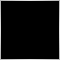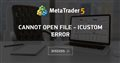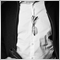# Question from a newbie code learner12

Dear Programmars

I am a super newbie who started coding about a week ago, trying to self-learn and make up an EA with my trading strategy base on Heikin Ashi and multi-time frame.

I was so happy to see my codes finally complies, however there are some error while running on the tester, such as similar problem like this https://www.mql5.com/en/forum/279663 and the trades did not go as how I wanted to be.

Doing my best to understand the mql language and modifing the codes from all sources online, here is my work along this week and hoping for somebody can point out anything that I might have missed or wrong in logic that caursed the errors.

Thank you so much for every helping hands, your reply will lead me to a big improvement. Thanks indeed.

```//+------------------------------------------------------------------+
//|                                                ForFreedom001.mq4 |
//|                                                                  |
//|                                                                  |
//+------------------------------------------------------------------+
#property copyright "ForFreedom001"
#property link      ""
#property version   "1.00"
#property strict
//+------------------------------------------------------------------+
//| Input                 宣告                                       |
//+------------------------------------------------------------------+
input string _="//--- General Setting ---//";
input int               Magic_number                         = 5678912;
input string            Open_Order_Comment                   = "ForFreedom001";
input int               Spread_Filter                        = 20;         // Spread_Filter (0=Disable)

input string __="//--- Entry Setting ---//";
input double            Position_One_Lot_Sizes               = 1.0;
input double            Position_Two_Lot_Sizes               = 0.8;
input double            Position_Three_Lot_Sizes             = 0.6;
input double            Position_Four_Lot_Sizes              = 0.4;
input double            Position_Five_Lot_Sizes              = 0.2;
input int               Max_Number_Of_Position               = 5;

input string ___="//--- Exit Setting ---//";
input int               Stop_Loss_In_Points                  = 400;

//+------------------------------------------------------------------+
//| Variables             自定義變數                                 |
//+------------------------------------------------------------------+

double Point_Value = 0;
int    Number_Of_Buy_Order = 0;
int    Number_Of_Sell_Order = 0;
double Open_Price_Of_Buy_Order = 0;
double Open_Price_Of_Sell_Order = 0;
bool   Close_Order_Status = true ;
int    Open_Order_Status = 0;

//+------------------------------------------------------------------+
//|       Functions             其他自定義變數                       |
//+------------------------------------------------------------------+

//----------------------------------------------------------------------------------------------------------BUY/SELL單一方向全部結單

void Close_Single_Direction_All_Orders(int Operation_Type)
{
for(int i=OrdersTotal()-1; i>=0; i--)// loop所有order

if(OrderSelect(i,SELECT_BY_POS,MODE_TRADES))//選擇訂單

if(OrderMagicNumber()==Magic_number && OrderSymbol()== Symbol() && OrderType()==Operation_Type)
(
Close_Order_Status=OrderClose(OrderTicket(),OrderLots(),OrderClosePrice(),0,Green) //結束
);
};

//------------------------------------------------------------------------------------------------------------查看LOT SIZE

double Trade_Lot_Size()
{
double Lot_Size = 0;

if(Number_Of_Buy_Order ==0 || Number_Of_Sell_Order ==0)
{Lot_Size = Position_One_Lot_Sizes;}
else if(Number_Of_Buy_Order ==1 || Number_Of_Sell_Order ==1)
{Lot_Size = Position_Two_Lot_Sizes;}
else if (Number_Of_Buy_Order ==2 || Number_Of_Sell_Order==2)
{Lot_Size = Position_Three_Lot_Sizes;}
else if (Number_Of_Buy_Order ==3 || Number_Of_Sell_Order==3)
{Lot_Size = Position_Four_Lot_Sizes;}
else if (Number_Of_Buy_Order ==4 || Number_Of_Sell_Order ==5)
{Lot_Size = Position_Five_Lot_Sizes;}
else Alert("Full Position");
return(Lot_Size);
}

//-------------------------------------------------------------------------------------------------------一支trend confirmation bar (4H)

bool NewBar_PERIOD_H4()
{
static datetime prevTime_H4    = 0;
datetime        currentTime_H4 = iTime(Symbol(),PERIOD_H4,0);

if(currentTime_H4!= prevTime_H4)
{
prevTime_H4=currentTime_H4;
return(true);
};
return(false);
};

//------------------------------------------------------------------------------------------------------- Heikin Ashi Value

double Prev_HA_Open = iCustom(NULL,PERIOD_H4,"heikinashi",2,1);
double Prev_HA_Close = iCustom(NULL,PERIOD_H4,"heikinashi",3,1);
double HA_Open = (Prev_HA_Open+Prev_HA_Close)/2;
double HA_Close = (iOpen(Symbol(),PERIOD_H4,1)+iHigh(Symbol(),PERIOD_H4,1)+iLow(Symbol(),PERIOD_H4,1)+iClose(Symbol(),PERIOD_H4,1))/4;
double Current_Low = iLow(Symbol(),PERIOD_H4,1);
double Current_High = iHigh(Symbol(),PERIOD_H4,1);

double HA_Low = MathMin(
MathMin(Current_Low,
HA_Open),
HA_Close
);

double HA_High = MathMax(
MathMin(Current_High,
HA_Open),
HA_Close
);

//+------------------------------------------------------------------+
//| Check_Opened_Orders                                              |
//+------------------------------------------------------------------+
void Check_Opened_Orders()
{

Number_Of_Buy_Order        =0;
Number_Of_Sell_Order       =0;
Open_Price_Of_Buy_Order    =0;
Open_Price_Of_Sell_Order   =0;

for(int i=0; i<OrdersTotal(); i++)
{
if(OrderSelect(i,SELECT_BY_POS,MODE_TRADES))
{
if(OrderMagicNumber()==Magic_number && OrderSymbol()==Symbol())
{
if(OrderType()==OP_BUY)
{
Number_Of_Buy_Order++;
Open_Price_Of_Buy_Order += OrderOpenPrice();
}
if(OrderType()==OP_SELL)
{
Number_Of_Sell_Order++;
Open_Price_Of_Sell_Order += OrderOpenPrice();
}
}
}
}
}

//+------------------------------------------------------------------+
//| Check_For_Close                                                  |
//+------------------------------------------------------------------+

//-----------------------------------------------------------------------------------------------------------查看BUY單是否符合條件平倉

void Check_For_Close()
{
if(Number_Of_Buy_Order>0)  //如果有BUY單
{
if(Number_Of_Buy_Order ==1 && Stop_Loss_In_Points>0 && Bid<=Open_Price_Of_Buy_Order-Stop_Loss_In_Points*Point_Value)  //----------- BUY單止蝕
{
Close_Single_Direction_All_Orders(OP_BUY); // Close 所有BUY單
}
else if(Number_Of_Buy_Order >=2 && Stop_Loss_In_Points>0 && Bid<=Open_Price_Of_Buy_Order-Stop_Loss_In_Points*Point_Value)
{
Close_Single_Direction_All_Orders(OP_BUY);
}
}
//-------------------------------------------------------------------------------------------------------------查看SELL單是否符合條件平倉
if(Number_Of_Sell_Order>0)  //如果有SELL單
{
if(Number_Of_Sell_Order ==1 && Stop_Loss_In_Points>0 && Ask>=Open_Price_Of_Sell_Order+Stop_Loss_In_Points*Point_Value) //----------- SELL 單止蝕
{
Close_Single_Direction_All_Orders(OP_SELL);  // ------------------------------------------------------Close 所有SELL單
}
else if( Number_Of_Sell_Order >=2 && Stop_Loss_In_Points>0 && Ask>=Open_Price_Of_Sell_Order+Stop_Loss_In_Points*Point_Value) //----------- SELL 單止蝕
{
Close_Single_Direction_All_Orders(OP_SELL); // End 如果有SELL單
}
}
} //Check_For_Close 完結

//+------------------------------------------------------------------+
//| Check_For_Open                                                   |
//+------------------------------------------------------------------+

void Check_For_Open()     //-----------------------------------------------------------------------查看户口是否符合條件開倉
{
if(OrdersTotal()<Max_Number_Of_Position)
{
if(NewBar_PERIOD_H4()) //------------------------------------------------------------------只是每一支4H HA bar 完成才run 一次
{
if(Spread_Filter>=MarketInfo(Symbol(), MODE_SPREAD) || Spread_Filter==0)  //---------------------Check Spread_Filter
{
if(HA_Open == HA_Low)  //在4H Heikin Ashi Chart, 上一支Bar 是沒有下影線
{
Open_Order_Status=OrderSend(Symbol(),OP_BUY,Trade_Lot_Size(),Ask,0,0,0,Open_Order_Status,clrGreen);
}

if(HA_Open == HA_High)
{
Open_Order_Status=OrderSend(Symbol(),OP_SELL,Trade_Lot_Size(),Bid,0,0,0,Open_Order_Status,clrRed);
}
} // End Spread Filter
return;} // End NewBar_PERIOD_4H
}
} //Check_For_Open 完結

//+------------------------------------------------------------------+
//| Expert initialization function                                   |
//+------------------------------------------------------------------+
int OnInit()
{
//---
Point_Value=MarketInfo(Symbol(),MODE_POINT); //------------計算這户口用的Point Value 是多少, 0.0001 還是0.00001
//---
return(INIT_SUCCEEDED);
}
//+------------------------------------------------------------------+
//| Expert deinitialization function                                 |
//+------------------------------------------------------------------+
void OnDeinit(const int reason)
{
//---

}
//+------------------------------------------------------------------+
//| Expert tick function                                             |
//+------------------------------------------------------------------+
void OnTick()
{
//---
Check_Opened_Orders();
Check_For_Close();
Check_For_Open();
}
//+------------------------------------------------------------------+

```Cannot Open File - iCustom Error
• 2018.09.17
• www.mql5.com
I am trying to return a value from an indicator buffer via iCustom, but I keep getting the error 'cannot open file': '2018.09.17 11:09:53...1273

Orders are orders. But you would probably want Positions....

Check the docs.
https://www.mql5.com/en/docs/trading

Another assumption you are doing:
iCustom does not return a value to be related to your usage!

https://www.mql5.com/en/docs/indicators/icustom

It's a handle.

Earliest call for iCustom should be in OnInit.

Do not init within global space.1273

A general hint for coding.

Warnings should be understood and not be ignored.